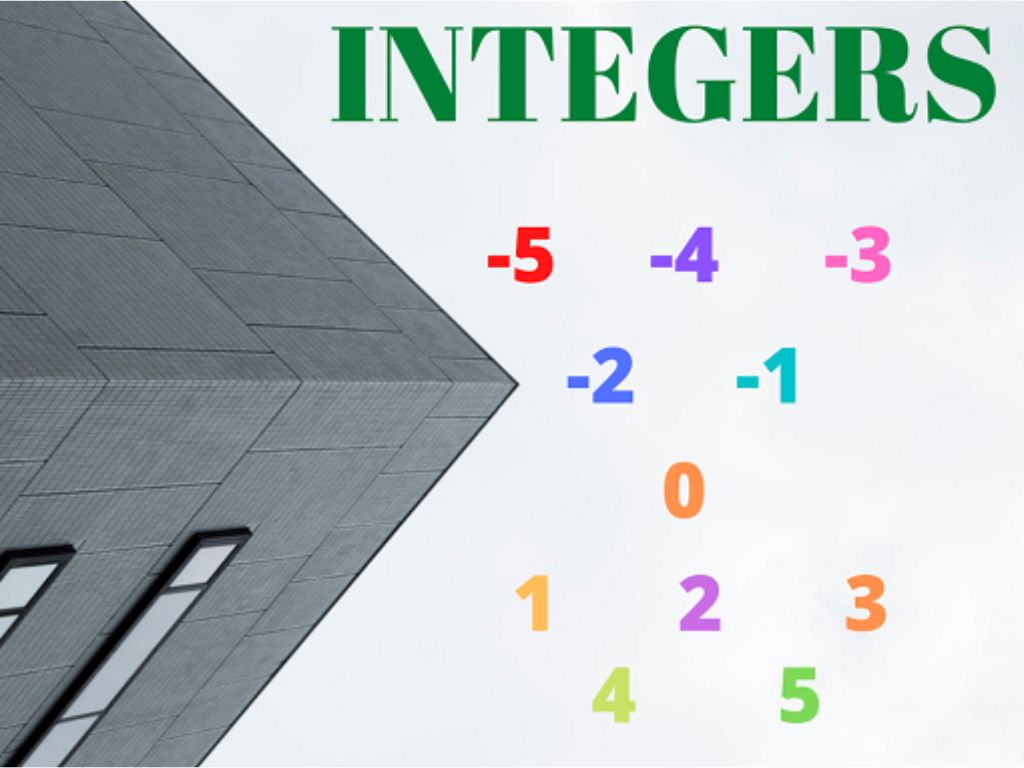## Integers Class 6th

Introduction In the number system, we have studied natural numbers and whole numbers. Now in class 6th, we shall study negative numbers also. We know that whole numbers are positive numbers along with zero (0). If we put positive numbers, negative numbers, and zero (0) together, the collection of these types of numbers is called …